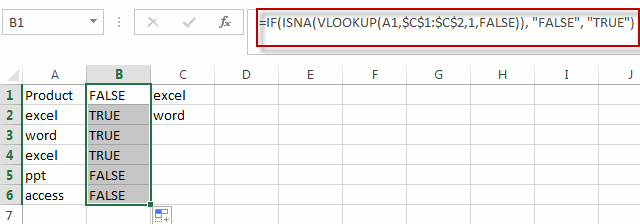Excel Vlookup Return True or False

This post will guide you how to vlookup values in a range of cells and then return True or False values in Excel. How to use the VLOOKUP function to vlookup a value in a list of data, and return True or False values in a selected cells.

In Excel, you can use the VLOOKUP function to look for a value in a column in a table and then returns TRUE from a given column in that table if it finds something. If it doesn’t, it returns FALSE.

Excel Vlookup Return True or False

Assuming that you have a list of data in range of cells A1:A6, and you want to search the range A1:A6 to look for values in range C1:C2. If found, then return True value, otherwise, return False. How to achieve it. You can use the VLOOKUP function in combination with the IF unction and the ISNA function to vlookup value. Like this:

=IF(ISNA(VLOOKUP(A1,\$C\$1:\$C\$2,1,FALSE)), "FALSE", "TRUE")

Type this formula in the formula box of Cell B1, and press Enter key in your keyboard. And drag the AutoFill handle over other cells to apply this formula.Related Functions

• Excel IF function
The Excel IF function perform a logical test to return one value if the condition is TRUE and return another value if the condition is FALSE. The IF function is a build-in function in Microsoft Excel and it is categorized as a Logical Function.The syntax of the IF function is as below:= IF (condition, [true_value], [false_value])….
• Excel VLOOKUP function
The Excel VLOOKUP function lookup a value in the first column of the table and return the value in the same row based on index_num position.The syntax of the VLOOKUP function is as below:= VLOOKUP (lookup_value, table_array, column_index_num,[range_lookup])….
• Excel ISNA function
The Excel ISNA function used to check if a cell contains the #N/A error, if so, returns TRUE; otherwise, the ISNA function returns FALSE.The syntax of the ISNA function is as below:=ISNA(value)….
Related Posts

VBA Macro For VLOOKUP From Another Sheet

In the previous post, you should know that how to fix or remove the #N/A error when using VLOOKUP formula to lookup value from another sheet. And this post will show you how to use VBA code to vlookup data ...

VLOOKUP From Another Sheet Not Working

In the previous post, you should know that how to fix or remove the #N/A error when using VLOOKUP formula to lookup value from another sheet. And this post will show you reasons why your VLOOKUP formula is not working ...

Fix #N/A Error For VLOOKUP From Another Sheet

This post will show you how to fix the #N/A error why it occurs when you extract values from another sheet using VLOOKUP function in Excel 2016,2013,2010 or other Excel versions. How can you correct a #N/A error in VLOOKUP ...

Get Employee Information by VLOOKUP

VLOOKUP is one of the key functions among all lookup & reference functions in Excel. Today, in this article, we will show you the way to apply VLOOKUP to retrieve employee information. I hope this article will help you in ...

VLOOKUP with Two Lookup Tables

VLOOKUP is one of the key functions among all lookup & reference functions in Excel. Today we will show you the application of VLOOKUP function when there are two lookup tables. EXAMPLE Table1 and table2 record the rates of Y2020 ...

VLOOKUP with Multiple Lookup Values

VLOOKUP is one of the key functions among all lookup & reference functions in Excel. It can scan and retrieve data from a static or dynamic table based on your lookup value. It can perform approximate match or exact match ...

VLOOKUP Data by Date

VLOOKUP is one of the key functions among all lookup & reference functions in Excel. It can scan and retrieve data from a static or dynamic table based on your lookup value. It can perform approximate match or exact match ...

VLOOKUP – Retrieve Data from Another Workbook

VLOOKUP is one of the key functions among all lookup & reference functions in Excel. It can scan and retrieve data from a static or dynamic table based on your lookup value. It can perform approximate match or exact match ...

VLOOKUP – Retrieve Data from Another Worksheet

VLOOKUP is one of the key functions among all lookup & reference functions in Excel. It can scan and retrieve data from a static or dynamic table based on your lookup value. It can perform approximate match or exact match ...

Case Sensitive Lookup with SUMPRODUCT and EXACT

Today, we will show you how to use SUMPRODUCT and EXACT to perform a case sensitive exact match. In this article, we provide a simple example to calculate bonus for employees whose names are case-sensitive. If you meet similar scenarios ...

Sidebar